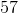# HSPT Math : How to do other word problems

## Example Questions

← Previous 1 3 4 5 6 7 8 9 10

### Example Question #471 : Problem Solving

What is the prime factorization of 48?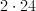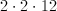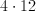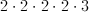Explanation:

We can find the prime factorization of a number by dividing 48 into smaller numbers.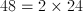2 is prime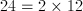2 is prime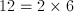2 is prime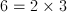Both 2 and 3 are prime.

So we have four 2's and one 3.  Multiply these numbers to get 48.

### Example Question #1 : How To Do Other Word Problems

What is the least common multiple of 6 and 8?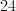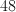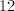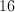Explanation:

One easy way to find the least common multiple is to take the largest of the numbers given to you, and find its multiples.

8: 8, 16, 24, 32, 40, 48...

Now start with 8, and see if any of these numbers is divisible by 6.  The first number that is divisible by 6 is the least common multiple of 6 and 8.

8: No

16: No

24: Yes

24 is the least common multiple of 6 and 8.

### Example Question #473 : Problem Solving

How many whole numbers are between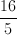and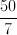?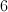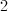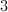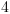Explanation:

Convert each fraction to a mixed number.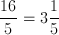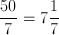So how many whole numbers are between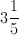and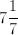?

4, 5, 6, and 7 would fall between these two numbers.  3 would not.  So there are 4 total whole numbers between them.

### Example Question #1 : How To Do Other Word Problems

What is the next number in this progression?

18, 10, 2, -6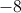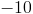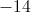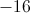Explanation:

This is an arithmetic progression. Therefore you must add or subtract a number to the number before it to arrive at the next number. We must find the difference between each number and apply it to the final number to find the next number in the series.

To start, take the second number and subtract the first number.

In this case it yields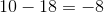Then take the third number and subtract the second number which results in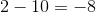If the resulting number is the same you now know the difference between each number in the sequence and can apply the difference to each resulting number.

In this case the difference isSo we take the final number and add the difference to it to result with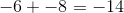### Example Question #1 : How To Do Other Word Problems

How many inches are in a foot?Explanation:

This is a conversion question.

There are 12 inches in a foot.

### Example Question #2 : How To Do Other Word Problems

What is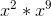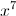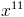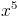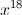Explanation:

When multiplying monomials with the same base, you add the powers of each monomial together.

So in this caseYielding an answer of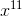### Example Question #3 : How To Do Other Word Problems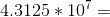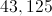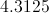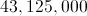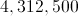Explanation:

This tests your knowledge of scientific notation.

To solve you must move the decimal places to the right to the power of the ten.

In this case you must move the decimal place to the right 7 spaces from its original spot.

The solution is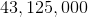### Example Question #2 : How To Do Other Word Problems

You have 5 types of meat, 3 different types of vegetables, and 8 different types of bread. How many different sandwiches can you make using only one of each ingredient?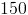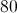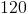Explanation:

This is a combination question.

To find the total number of sandwiches using only one ingredient we create three spaces for each ingredient and place each number of possible ingredients into each space.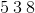We then multiply each number in each space together to find the answer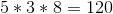### Example Question #3 : How To Do Other Word Problems

The square of what number is 75 divided by 3?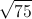Explanation:

To answer this question we must first divide the original number by the number following it to get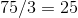Once we have this number we then square root it to find the answer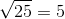### Example Question #6 : How To Do Other Word Problems

What is the absolute value of -57?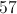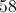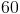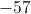In this case the answer is